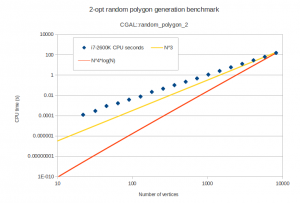# Random polygons with CGAL

At some point I'm going to need random simple polygons for testing my voronoi diagram code. CGAL includes a function random_polygon_2 which uses the "2-opt" heuristic (see Auer&Held-1996).

I wrote a minimal boost::python wrapper around this function in order to test it and draw the polygons using vtk. Here are some examples of random polygons with randomly placed vertices inside the unit circle:

The one with 8k vertices takes 150 seconds of i7-2600K CPU time to generate. The worst-case running time of this algorithm is slow, O(n^4 log n), but it practice it seems better than O(n^3):This site uses Akismet to reduce spam. Learn how your comment data is processed.